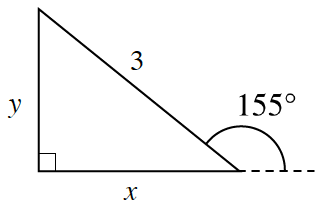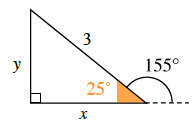### Home > INT3 > Chapter 2 > Lesson 2.1.2 > Problem2-20

2-20.

A $3$-foot indoor children’s slide must meet the ground very gradually and make an angle of $155^\circ$, as shown in the diagram at right. What are the height of the slide ($y$) and the length of the floor it will cover ($x$)?Review the trigonometric ratios from the Math Notes box in this lesson. Which angle represents "?" for those equations?

In order to produce the correct value, the inside angle must be used. See the diagram.